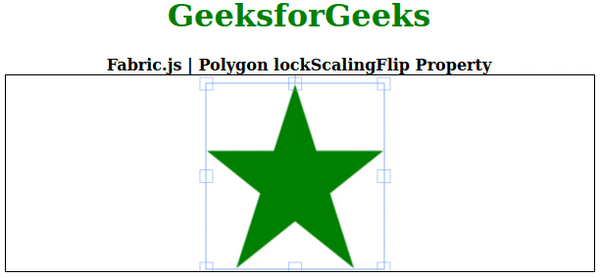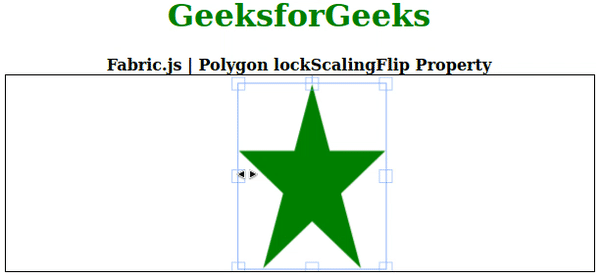# Fabric.js Polygon lockScalingFlip Property

• Last Updated : 26 Aug, 2020

In this article, we are going to see how to lock flipping by scaling a canvas polygon using FabricJS so that it cannot be inverted by pulling the width to the negative side. The canvas polygon means the polygon is movable and can be stretched according to requirement. Further, the polygon can be customized when it comes to initial stroke color, height, width, fill color, or stroke width.

To make it possible we are going to use a JavaScript library called FabricJS. After importing the library, we will create a canvas block in the body tag that will contain the polygon. After this, we will initialize instances of Canvas and polygon provided by FabricJS and lock flipping by scaling of the polygon using lockScalingFlip property and render the polygon on the Canvas as given in the example below.

Syntax:

```fabric.Polygon([
{ x: pixel, y: pixel },
{ x: pixel, y: pixel },
{ x: pixel, y: pixel},
{ x: pixel, y: pixel},
{ x: pixel, y: pixel }],
{
lockScalingFlip: boolean
});
```

Parameter: This property accepts a single parameter as mentioned above and described below:

• lockScalingFlip: It specifies whether to lock flipping by scaling or not.

Note: Dimension pixels is must create a polygon.

Below examples illustrate the Fabric.JS Polygon lockScalingFlip property in JavaScript:

Example 1:

## HTML

 ` ``<``html``> `` ` `<``head``> ``    ````    ``<``script` `src``= ``"https://cdnjs.cloudflare.com/ajax/libs/fabric.js/3.6.2/fabric.min.js"``> ``    `` `` `` ` `<``body``> ``    ``<``div` `style``=``"text-align: center;width: 600px;"``> ``    ``<``h1` `style``=``"color: green;"``> ``        ``GeeksforGeeks ``    `` ``    ``<``b``> ``        ``Fabric.js | Polygon lockScalingFlip Property ``    `` ``    `` ``    ``<``canvas` `id``=``"canvas"``            ``width``=``"600"``            ``height``=``"200"``            ``style``=``"border:1px solid #000000;"``> ``    `` `` ` `    ``<``script``> `` ` `        ``// Initiate a Canvas instance ``        ``var canvas = new fabric.Canvas("canvas"); `` ` `        ``// Initiate a polygon instance ``        ``var polygon = new fabric.Polygon([ ``        ``{ x: 295, y: 10 }, ``        ``{ x: 235, y: 198 }, ``        ``{ x: 385, y: 78}, ``        ``{ x: 205, y: 78}, ``        ``{ x: 355, y: 198 }], { ``            ``fill: 'green',``            ``lockScalingFlip: true``        ``}); `` ` `        ``// Render the polygon in canvas ``        ``canvas.add(polygon); ``    `` `` `` ` ``

Output:Example 2:

## HTML

 ` ``<``html``> `` ` `<``head``> ``    ````    ``<``script` `src``= ``"https://cdnjs.cloudflare.com/ajax/libs/fabric.js/3.6.2/fabric.min.js"``> ``    `` `` `` ` `<``body``> ``    ``<``div` `style``=``"text-align: center;width: 600px;"``> ``    ``<``h1` `style``=``"color: green;"``> ``        ``GeeksforGeeks ``    `` ``    ``<``b``> ``        ``Fabric.js | Polygon lockScalingFlip Property ``    `` ``    `` ``    ``<``canvas` `id``=``"canvas"``            ``width``=``"600"``            ``height``=``"200"``            ``style``=``"border:1px solid #000000;"``> ``    `` `` ` `    ``<``script``> `` ` `        ``// Initiate a Canvas instance ``        ``var canvas = new fabric.Canvas("canvas"); `` ` `        ``// Initiate a polygon instance ``        ``var polygon = new fabric.Polygon([ ``        ``{ x: 295, y: 10 }, ``        ``{ x: 235, y: 198 }, ``        ``{ x: 385, y: 78}, ``        ``{ x: 205, y: 78}, ``        ``{ x: 355, y: 198 }], { ``            ``fill: 'green',``            ``lockScalingFlip: false``        ``}); `` ` `        ``// Render the polygon in canvas ``        ``canvas.add(polygon); ``    `` `` `` ` ``

Output:My Personal Notes arrow_drop_up